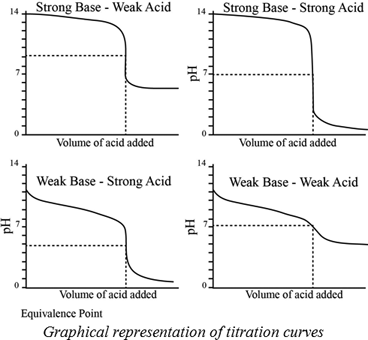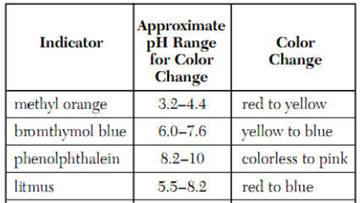# Without doing detailed calculations, sketch the curve for the titration of 30.0 mL of 0.10 M NaOH with 0.10 M HCl. Indicate the approximate pH at the beginning of the titration and at the equivalence point. What is the total solution volume at the equivalence point?### Chemistry & Chemical Reactivity

9th Edition
John C. Kotz + 3 others
Publisher: Cengage Learning
ISBN: 9781133949640

#### Solutions

Chapter
Section### Chemistry & Chemical Reactivity

9th Edition
John C. Kotz + 3 others
Publisher: Cengage Learning
ISBN: 9781133949640
Chapter 17, Problem 31PS
Textbook Problem
116 views

## Without doing detailed calculations, sketch the curve for the titration of 30.0 mL of 0.10 M NaOH with 0.10 M HCl. Indicate the approximate pH at the beginning of the titration and at the equivalence point. What is the total solution volume at the equivalence point?

Interpretation Introduction

Interpretation:

The curve for titration of 30.0 mL of 0.10 M NaOH with 0.10 M HCl has to be sketched. The approximate pH at the beginning of the titration and at a equivalence point has to be indicated. And the total solution volume at the equivalence point has to be determined.

Concept introduction:

A titration is one of the most useful ways of determining accurately the quantity of an acid , a base or some other substances in a mixture.

The pH at the equivalence point of a strong acid –strong base titration is 7.

A weak acid titrated with a  strong base leads to a pH > 7 at the equivalence point.

A weak base titrated with a strong acid leads to pH < 7 at the equivalence point.Indicators:

A chemical substances which gives a visible change  in the titration.

Some important indicators are as follow.### Explanation of Solution

1.0 M NaOH of pH is 14 and because of pH is logarithmic, a 1.0 M NaOH solution pH will be 13.

Here, we are using for titration acidic solution versus basic solution. Therefore, acidic solution will be acidic at high concentrations NaOH is strong bas and HCl is a strong acid. Therefore, the equivalence point at pH=7

### Still sussing out bartleby?

Check out a sample textbook solution.

See a sample solution

#### The Solution to Your Study Problems

Bartleby provides explanations to thousands of textbook problems written by our experts, many with advanced degrees!

Get Started

Find more solutions based on key concepts
This controversy addresses accusations launched against sugars in foods and beverages as causes of health probl...

Nutrition: Concepts and Controversies - Standalone book (MindTap Course List)

Distinguish between acids and bases. What are their properties?

Biology: The Dynamic Science (MindTap Course List)

The correct structural for ethyne is: a. HC=CH b. HC=C=H c. HCCH d. H=C=C=H

Chemistry for Today: General, Organic, and Biochemistry

It has been suggested dial rotating cylinders about 20 km in length and 8 km in diameter be placed in space and...

Physics for Scientists and Engineers, Technology Update (No access codes included)

What are water masses? What determines their relative position in the ocean?

Oceanography: An Invitation To Marine Science, Loose-leaf Versin

How does astronomy help answer the question, What are we?

Foundations of Astronomy (MindTap Course List)